# Exploring Variable-Step Solvers Using a Stiff Model

This example shows the behaviour of variable-step solvers in a Foucault pendulum model. Simulink® solvers ode45, ode15s, ode23, and ode23t are used as test cases. Stiff differential equations are used to solve this problem. There is no exact definition of stiffness for equations. Some numerical methods are unstable when used to solve stiff equations and very small step-sizes are required to obtain a numerically stable solution to a stiff problem. A stiff problem may have a fast changing component and a slow changing component.

Foucault pendulum is an example of a stiff problem. The pendulum completes an oscillation in a few seconds (fast changing component) whereas the Earth completes a rotation about its axis in a day (slow changing component). The oscillation plane of the pendulum slowly rotates because of Earth's axial rotation. Read more about the physics of a Foucault pendulum in Modelling a Foucault Pendulum.

The simulation calculates the position of the pendulum bob in the x-y plane as viewed by an observer on the surface of Earth. The total energy of the pendulum is then calculated and used to assess the performance of various Simulink solvers.

### Foucault Pendulum Model

The Foucault pendulum is described by the system of coupled differential equations given below. Friction and air drag are not taken into consideration (this greatly simplifies the equations). A full derivation of these equations is given in the Foucault Pendulum example.The model shown in Figure 1 is used to solve the differential equations describing a Foucault pendulum. Type sldemo_solvers in MATLAB® Command Window to open the model. The example simulates a Foucault pendulum for 86400 seconds. The constants and initial conditions are saved the model workspace.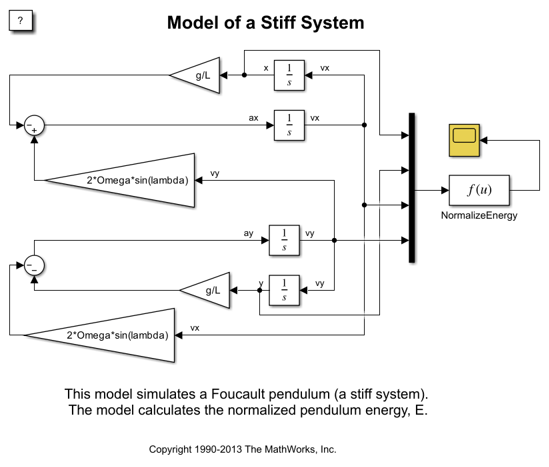Figure 1: Foucault pendulum model used top assess solver performance

### Variable Step Solvers

This example investigates the performance of ode45, ode15s, ode23, and ode23t variable-step solvers. To read more about a particular solver, for example ode45, type help ode45 in MATLAB Command Window.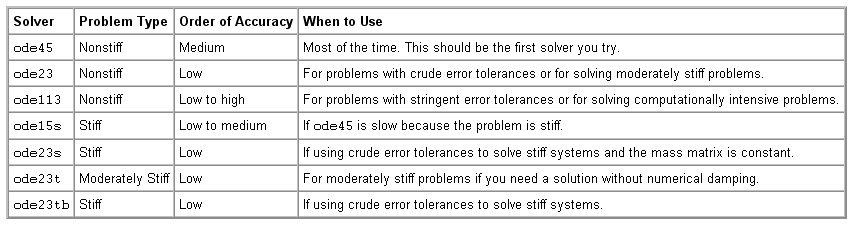Figure 2: List of variable-step solvers available in Simulink

### Assessing Solver Performance

There are different ways to assess the performance of a solver. If a problem has a closed form solution, you could compare the solver results with the expected theoretical result. You could also monitor the time it takes to simulate a model using a particular solver.

Unfortunately there is no exact theoretical solution for the Foucault Pendulum problem. There is an approximate closed form solution, however it cannot be used to assess solver performance (see the Foucault pendulum example).

### Total Energy Conservation

This example assesses solver performance by verifying the energy conservation law. In a frictionless environment, the total energy of the pendulum must remain constant. The calculated energy of the pendulum, however, will not remain constant as a result of limited numeric accuracy.

This example calculates the normalized total energy of the pendulum at every time-step. The relative error in energy equals the change in total energy over the course of the simulation. Ideally, the relative error in energy must be zero because energy is conserved. Total energy is the sum of potential and kinetic energy. "NormalizeEnergy" block calculates normalized pendulum energy using the expressions given below.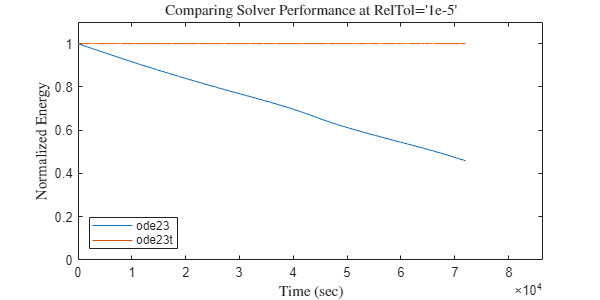Figure 3: Normalized energy versus time

Figure 3 shows a plot of normalized energy versus time as calculated using ode23 and ode23t. It is clear that in this particular problem ode23t is much more accurate than ode23. In the simulation that used ode23, the normalized pendulum energy decreased by more than 60%. A pendulum with lower energy has a lower oscillation amplitude. You can see this effect in Figure 4, where the amplitude of the pendulum calculated by ode23 decreases as the oscillation plane rotates.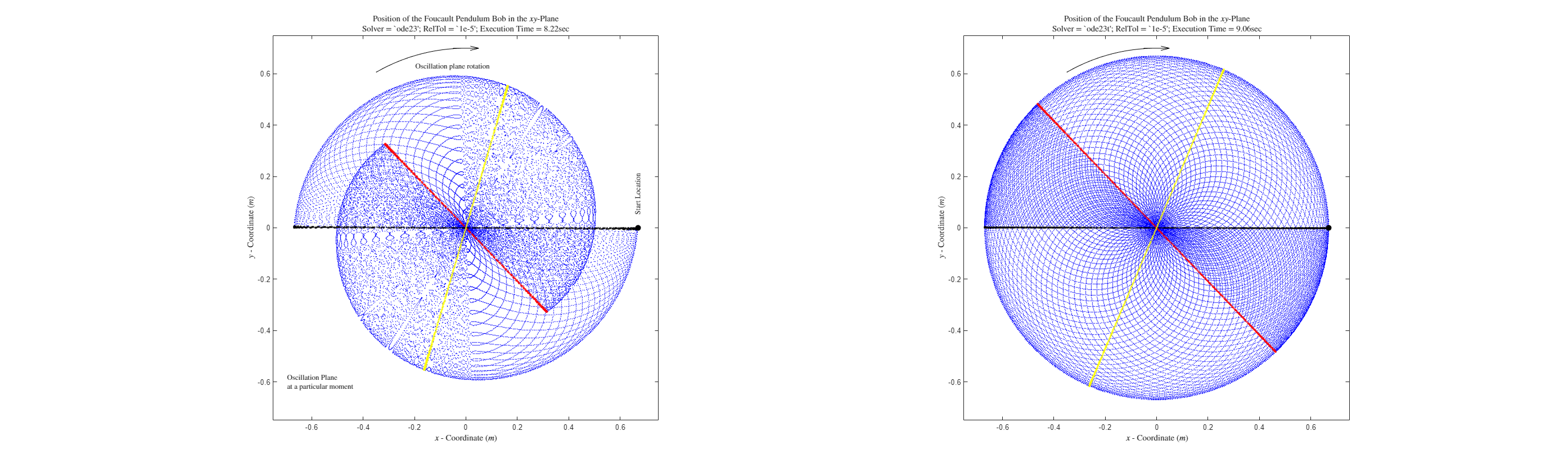Figure 4: Pendulum position as calculated using ode23 and ode23t

Figure 4 illustrates the difference between a stiff and a non-stiff solver. Each plot shows the position of the pendulum bob throughout a day (every 15th data point is plotted as a blue point). The black dot marks the initial position of the pendulum bob and the black line marks the initial pendulum oscillation plane. The red line indicates the oscillation plane after a day. The yellow line shows the oscillation plane at some intermediate point in time. Note that the oscillation plane of the pendulum does not complete a full rotation within a day. How fast the oscillation plane rotates depends on the geographical latitude (see details in the Foucault pendulum example). Observe that the pendulum amplitude in the left plot decreases whereas the amplitude in the right plot remains constant. For the same relative tolerance, RelTol=1e-5, the stiff solver gives a more accurate result, but requires more execution time.

Figure 5 shows a more detailed performance study of Simulink solvers. Four solvers were chosen to illustrate how relative error and simulation execution time vary as a function of relative tolerance. You can use the sldemo_solvers_mcode.m script for more extensive solver tests. This script generates C code from the model to speed up the simulations. Note that the script can take several minutes to execute.

Building RSim executable for model.. Time taken: 22.775988 seconds. Running generated RSim executable.. Time taken: 272.104904 seconds.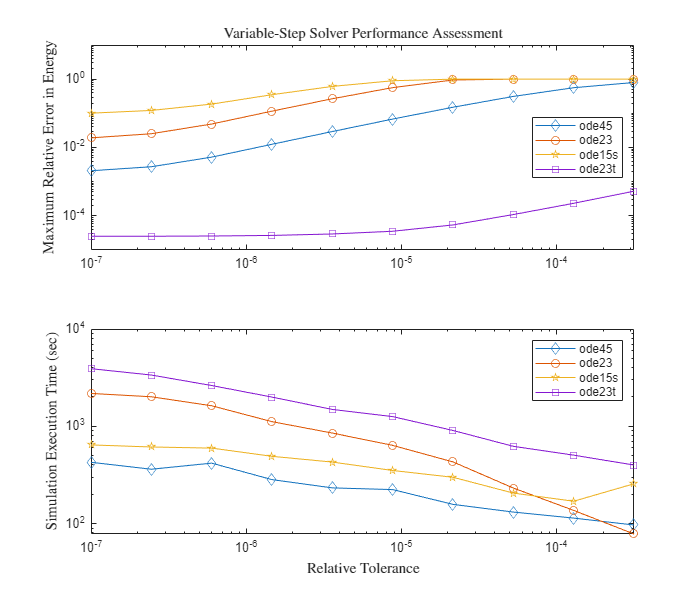Figure 5: Relative error and execution time for various solver settings. AbsTol = '1e-7' for all simulations.

Observe that in this example relative error does not decrease very significantly for relative tolerances below 1e-6. This is a numeric solver limitation that depends on the particular model. Reducing the solver relative tolerance does not necessarily improve accuracy. You need to estimate the minimal accuracy that is required for your problem and choose the solver accordingly to minimize simulation costs. For example you may want to know the position of the Foucault pendulum bob within a few centimeters. It is unnecessary to calculate the position of a pendulum within a few microns because you cannot measure the position that accurately.

### Conclusions

The numeric results of a simulation can behave differently depending on solver settings. This is crucial in the case of stiff problems. When working with stiff models, choose a solver that will give an accurate result at a minimal price. The relative tolerance (variable-step solvers) or step size (fixed-step solvers) have to be small enough that the result is numerically stable. Although some solvers are more efficient than others, stiff solvers are better suited for solving stiff problems. Variable step solvers are more robust than fixed-step solvers.